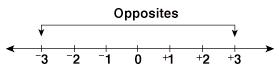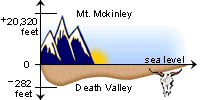# Subtracting Integers

## Learn About Subtracting Integers With The Following Integer Examples And Interactive ExercisesProblem: The temperature in Anchorage, Alaska was 8°F in the morning and dropped to -5°F in the evening. What is the difference between these temperatures?

Solution: We can solve this problem using integers. Using the number line below, the distance from +8 to 0 is 8, and the distance from 0 to -5 is 5, for a total of 13.

+8 - -5 =  +13. The difference is 13 degrees.Problem: The highest elevation in North America is Mt. McKinley, which is 20,320 feet above sea level. The lowest elevation is Death Valley, which is 282 feet below sea level. What is the difference between these two elevations?

Solution: When solving problems with large integers, it is not always practical to rely on the number line. Using integer arithmetic this problem becomes:

+20,320 - -282 = ?

We need a rule for subtracting integers in order to solve this problem.

Rule: To subtract an integer, add its opposite.

The opposite of -282 is +282, so we get: +20,320 - -282 = +20,320 + +282 = +20,602

In the above problem, we added the opposite of the second integer and subtraction was transformed into addition. Let's look at some simpler examples of subtracting integers.

Example 1: +5 - +2Step 1: The opposite of +2 is -2.

Solution: +5 - +2 = +5 + -2 = +3

Example 2: Find the difference between each pair of integers.Subtracting Integers Subtract Add The Opposite Result +9 - +4 = +9 + -4 = +5 +9 - -4 = +9 + +4 = +13 -9 - +4 = -9 + -4 = -13 -9 - -4 = -9 + +4 = -5

Notice that in each problem above, the first integer remained unchanged. Also, do not confuse the sign of the integer with the operation being performed. Remember that:
-9 + +4 = -5   is read as Negative 9 plus positive 4 equals negative 5.

Let's look at some more examples:

Example 3: Find the difference between each pair of integers.Subtracting Integers Subtract Add The Opposite Result +7 - +10 = +7 + -10 = -3 +7 - -10 = +7 + +10 = +17 -7 - +10 = -7 + -10 = -17 -7 - -10 = -7 + +10 = +3

Example 4: Find the difference between each pair of integers. You may extend the number line below to help you salve these problems.Subtracting Integers Subtract Add The Opposite Result -8 - +3 = -8 + -3 = -11 +17 - -9 = +17 + +9 = +26 -12 - +15 = -12 + -15 = -27 -19 - -23 = -19 + +23 = +4

Summary: To subtract an integer, add its opposite. When subtracting integers, it is important to show all work, as we did in this lesson. If you skip steps, or do the work in your head, you are very likely to make a mistake--even if you are a top math student!### Exercises

Directions: Read each question below. Click once in an ANSWER BOX and type in your answer; then click ENTER. After you click ENTER, a message will appear in the RESULTS BOX to indicate whether your answer is correct or incorrect. To start over, click CLEAR.

 1.+6 - +9 = ? ANSWER BOX:   RESULTS BOX:
 2.+11 - -8 = ? ANSWER BOX:   RESULTS BOX:
 3.-13 - +5 = ? ANSWER BOX:   RESULTS BOX:
 4.-2 - -15 = ? ANSWER BOX:   RESULTS BOX:
 5.-9 - +14 = ? ANSWER BOX:   RESULTS BOX: